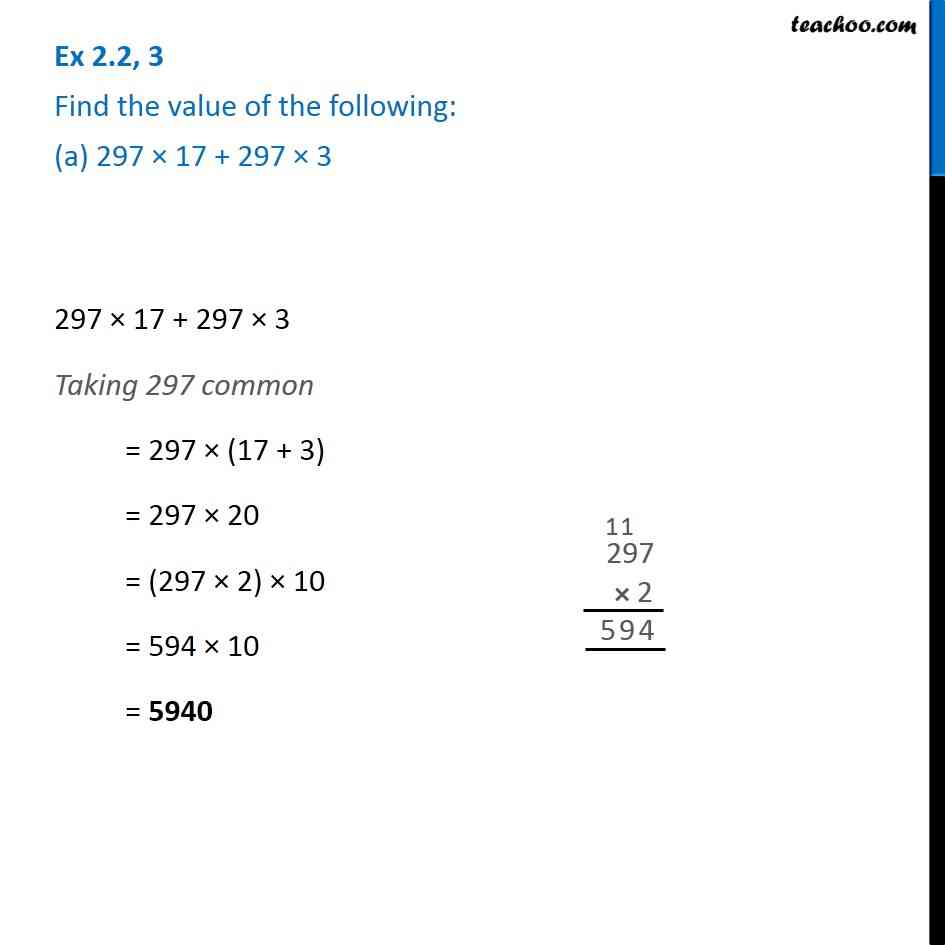1. Chapter 2 Class 6 Whole Numbers
2. Concept wise
3. Distributivity

Transcript

Ex 2.2, 3 Find the value of the following: (a) 297 × 17 + 297 × 3 297 × 17 + 297 × 3 Taking 297 common = 297 × (17 + 3) = 297 × 20 = (297 × 2) × 10 = 594 × 10 = 5940